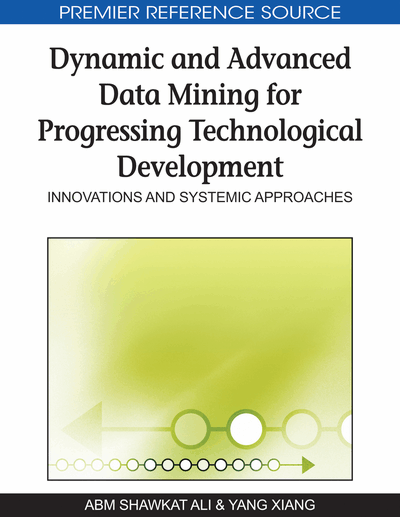# Financial Data Mining Using Flexible ICA-GARCH Models

Philip L.H. Yu (The University of Hong Kong, Hong Kong), Edmond H.C. Wu (The Hong Kong Polytechnic University, Hong Kong) and W.K. Li (The University of Hong Kong, Hong Kong)
DOI: 10.4018/978-1-60566-908-3.ch011
Available
\$37.50
No Current Special Offers

## Abstract

As a data mining technique, independent component analysis (ICA) is used to separate mixed data signals into statistically independent sources. In this chapter, we apply ICA for modeling multivariate volatility of financial asset returns which is a useful tool in portfolio selection and risk management. In the finance literature, the generalized autoregressive conditional heteroscedasticity (GARCH) model and its variants such as EGARCH and GJR-GARCH models have become popular standard tools to model the volatility processes of financial time series. Although univariate GARCH models are successful in modeling volatilities of financial time series, the problem of modeling multivariate time series has always been challenging. Recently, Wu, Yu, & Li (2006) suggested using independent component analysis (ICA) to decompose multivariate time series into statistically independent time series components and then separately modeled the independent components by univariate GARCH models. In this chapter, we extend this class of ICA-GARCH models to allow more flexible univariate GARCH-type models. We also apply the proposed models to compute the value-at-risk (VaR) for risk management applications. Backtesting and out-of-sample tests suggest that the ICA-GARCH models have a clear cut advantage over some other approaches in value-at-risk estimation.
Chapter Preview
Top

## Volatility Models

In the following, we introduce several prevailing volatility models for financial time series.

### GARCH (p,q) Model

In GARCH models, financial time series {yt} are assumed to be generated by a stochastic process with (conditional) time-varying volatility {σt}. The general GARCH (p, q) model ( p > 0 and q ≥ 0 are integers) is defined as

(1)where α0 > 0, αi ≥ 0 forand βj ≥ 0 for, and D(0,1) represents a conditional distribution with zero mean and unit variance such as the standard Gaussian distribution N(0,1) and the Student's t distribution (standardized to unit variance). Since GARCH(1,1) model was found to be adequate for many financial time series (Bollerslev, Chou, & Kroner, 1992), we focused on this model in our empirical analysis.

## Complete Chapter List

Search this Book:
Reset